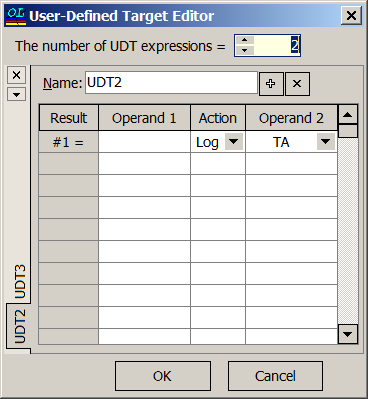### User-Defined Targets

 User-Defined Target (UDT) can be an arbitrary expression that includes all available OptiLayer's elementary targets (like RS, TS, etc.), constants, previously defined (intermediate) targets and arithmetic operators. It can also include elementary functions: trigonometric functions Sin(), Cos(), exponent Exp(), hyperbolic functions Sh(), Ch(), Th(), absolute value Abs(). Design example: Polarizator with $$T_p$$>98% and $$T_p/T_s>200$$ in the spectral range from 780 nm to 820 nm, incidence angle is 45 degrees. In this case two targets are to be specified: a range target ($$T_p>98%$$) and the user-defined target shown on the right panel. The combination of these two targets is shown below: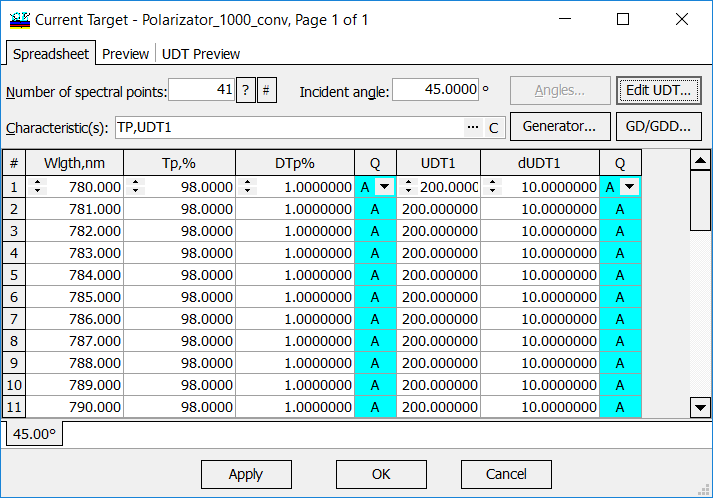The letter "A" (Above) in Q (Qualifiers) column stands for range requirement: $$T_p>98\%$$ and $$UDT1=T_p/T_s>200$$. UDT is constructed starting from the Result #1 row to the last Result row of the User-Defined Target Editor. In the example below: $UDT1=\frac{T_p}{T_s}$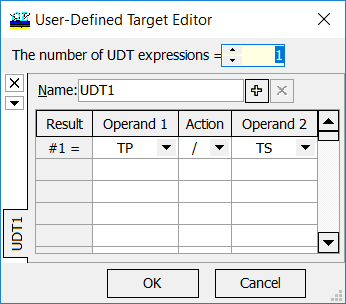A polarizator exhibiting excellent spectral performance  can be obtained by OptiLayer in a few seconds. User-defined targets can be used in the course of solving of many design problems, for example, multilayer polarizers or polarizing beamsplitters. Transmittance (s- and p-polarization) of one of the designed thin-film polarizators: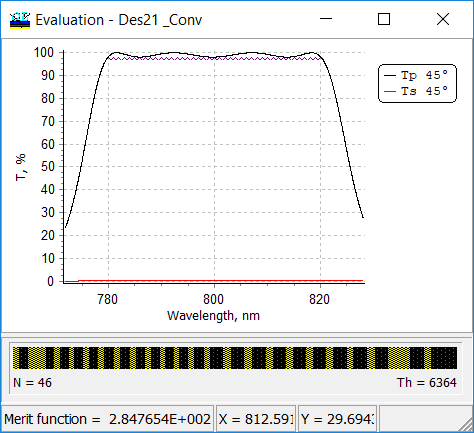OptiLayer allows you to plot the extinction ration $$T_p/T_s$$ is plotted in another window: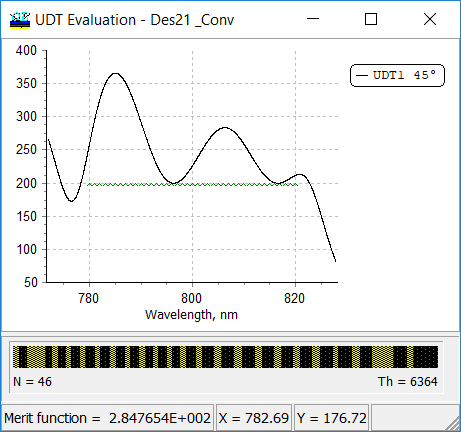Starting from 11.03 version, more functions are added. These function allow you to specify even more sophisticated targets: Sqrt - square root, Cbrt - cubic root, Log - logarithm, Lg10 - base-10 logarithm, Tan - tangent, ASin - arcsin, ACos - arccos, ATan - arctan,  Erf - error function, ASinh - hyperbolic arcsin, ACosh - hyperbolic arccos, ATanh - hyperbolic arctan.